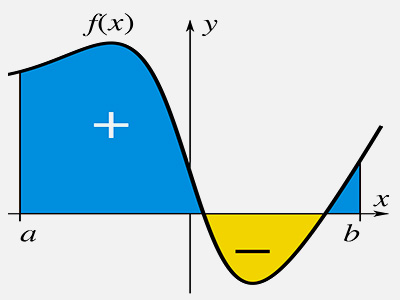ΓραμματείαSecretariat: 2410 684574 | ΦοιτητικάStudents: 2410 684387 g-ds@uth.gr
Select Page

# Numerical Analysis

Υ301

3

#### Hours/Week - ECTS

5 – 5### Learning Outcomes

The course aims to give students the necessary tools to solve known mathematical problems that arise in computer science and telecommunications, such as solving linear systems, differential and non-linear equations, data access problems etc.). The use of MATLAB makes it possible to implement and study the methods presented in the theory.

After the successful completion of the course students will be able:

• to understand ways to solve linear systems with immediate and repetitive methods and judge which is the appropriate method to use in each problem.
• to understand the basic methods of solving nonlinear systems of equations.
• to understand the methods of approaching and interpolating data with (in parts) polynomial and/or trigonometric functions (Fourier).
• to understand the basic methods of numerical derivation with finite differences and integration, which will be useful in solving differential equations with numerical methods.
• to understand the effect of finite arithmetic errors and method errors on the arithmetic results that will be obtained from the execution of the programmed methods.
• to use MATLAB its tools (toolbox).

### Indicative Module Content

• Computer Arithmetic
• Solving Nonlinear Equations
• Solving Systems of Linear and Nonlinear Equations
• Selected Topics in Numerical Linear Algebra
• Approximating Functions
• Numerical Differentiation and Integration
• Numerical Solution of Ordinary Differential Equations
• Numerical Solution of Partial Differential Equations
• An Overview of Mathematical Software (MATLAB)